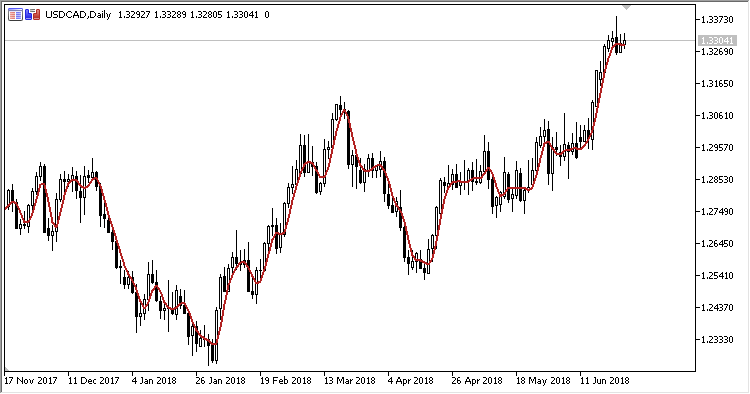# FIR – indicator for MetaTrader 5

• A+
category：MT5 INDICATORS

Indicator FIR is a symmetrically weighted filter (Finite Impulse Response Filter).

It has one input parameter:

• Applied price - price used for calculations.

Calculations:

`FIR = (Price(0) + 2*Price(1) + 2*Price(2) + Price(3)) / 6`

where:

`Price = SMA(1, Applied price)`